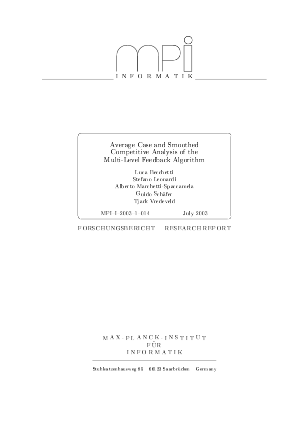Document# Average Case and Smoothed Competitive Analysis of the Multi-Level Feedback Algorithm

### Authors Luca Becchetti, Stefano Leonardi, Alberto Marchetti-Spaccamela, Guidouca Schaefer, Tjark Vredeveld## Files

DagSemProc.05031.7.pdf
• Filesize: 427 kB
• 35 pages

## Cite As

Luca Becchetti, Stefano Leonardi, Alberto Marchetti-Spaccamela, Guidouca Schaefer, and Tjark Vredeveld. Average Case and Smoothed Competitive Analysis of the Multi-Level Feedback Algorithm. In Algorithms for Optimization with Incomplete Information. Dagstuhl Seminar Proceedings, Volume 5031, pp. 5-28, Schloss Dagstuhl - Leibniz-Zentrum für Informatik (2005)
https://doi.org/10.4230/DagSemProc.05031.7

## Abstract

In this paper we introduce the notion of smoothed competitive analysis of online algorithms. Smoothed analysis has been proposed by Spielman and Teng to explain the behaviour of algorithms that work well in practice while performing very poorly from a worst case analysis point of view. We apply this notion to analyze the Multi-Level Feedback (MLF) algorithm to minimize the total flow time on a sequence of jobs released over time when the processing time of a job is only known at time of completion. The initial processing times are integers in the range $[1,2^K]$. We use a partial bit randomization model, i.e., the initial processing times are smoothed by changing the $k$ least significant bits under a quite general class of probability distributions. We show that MLF admits a smoothed competitive ratio of $O((2^k/\psigma)^3 + (2^k/\psigma)^2 2^{K-k}})$, where $\sigma$ denotes the standard deviation of the distribution. In particular, we obtain a competitive ratio of $O(2^{K-k})$ if $\sigma = \Theta(2^k)$. We also prove an $\Omega(2^{K-k})$ lower bound for any deterministic algorithm that is run on processing times smoothed according to the partial bit randomization model. For various other smoothing models, including the additive symmetric smoothing one, which is a variant of the model used by Spielman and Teng, we give a higher lower bound of $\Omega(2^K)$. A direct consequence of our result is also the first average case analysis of MLF. We show a constant expected ratio of the total flow time of MLF to the optimum under several distributions including the uniform one.
##### Keywords
• Competitive analysis
• average case analysis
• smoothed analysis
• scheduling

## Metrics

• Access Statistics
• Total Accesses (updated on a weekly basis)
0